# Clock hands

Calculate the internal angles of a triangle whose vertices lie on the clock's 2, 6 and 11 hours.

A =  75 °
B =  60 °
C =  45 °

### Step-by-step explanation:

$B=\alpha \mathrm{/}2=120\mathrm{/}2=60\text{°}$
$C=\gamma \mathrm{/}2=90\mathrm{/}2=45\text{°}$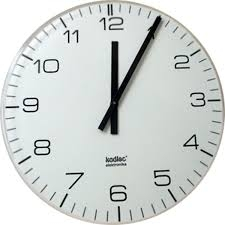Did you find an error or inaccuracy? Feel free to write us. Thank you!Tips to related online calculators
Do you want to convert time units like minutes to seconds?

#### You need to know the following knowledge to solve this word math problem:

We encourage you to watch this tutorial video on this math problem:

## Related math problems and questions:

• Clock hands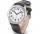The hands-on clock shows the time 12 hours and 2 minutes. Calculate the size of an acute angle between clock hands three hours later.
• Interior angles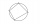In a quadrilateral ABCD, whose vertices lie on some circle, the angle at vertex A is 58 degrees and the angle at vertex B is 134 degrees. Calculate the sizes of the remaining interior angles.
• Clock Tower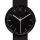What angle is betwenn hands on Clock Tower when show 17 hours and 35 minutes?
• Clock faceclock face is given. Numbers 10 and 5, and 3 and 8 are connected by straight lines. Calculate the size of their angles.
• Circumferential angleVertices of the triangle ΔABC lay on the circle and divided into arcs in the ratio 7:8:7. Determine the size of the angles of the triangle ΔABC.
• HandsThe clock shows 12 hours. After how many minutes will angle between the hour and minute hand 90°? Consider the continuous movement of both hands hours.
• Internal and external anglesCalculate the remaining internal and external angles of a triangle, if you know the internal angle γ (gamma) = 34 degrees and one external angle is 78 degrees and 40 '. Determine what kind of triangle it is from the size of its angles.
• Triangle anglesThe angles α, β, γ in triangle ABC are in the ratio 6:2:6. Calculate size of angles.
• Smallest internal angle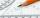Calculate what size has the smallest internal angle of the triangle if values of angles α:β:γ = 3:4:8
• Inscribed triangle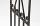To a circle is inscribed triangle so that the it's vertexes divide circle into 3 arcs. The length of the arcs are in the ratio 2:3:7. Determine the interior angles of a triangle.
• Internal anglesFind the internal angles of the triangle ABC if the angle at the vertex C is twice the angle at the B and the angle at the vertex B is 4 degrees smaller than the angle at the vertex A.
• The chordThe side of the triangle inscribed in a circle is a chord passing through the circle center. What size are the internal angles of a triangle if one of them is 40°?
• Little hand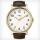What angle shifted little hand on the clock after one hour and 38 minutes?
• AnglesThe triangle is one outer angle 158°54' and one internal angle 148°. Calculate the other internal angles of a triangle.
• DiagonalsDraw a square ABCD whose diagonals have a length of 6 cm
• ClockHow many times a day hands on a clock overlap?
• Angles in triangleThe triangle is ratio of the angles β:γ = 6:8. Angle α is 40° greater than β. What are the size of angles of the triangle?